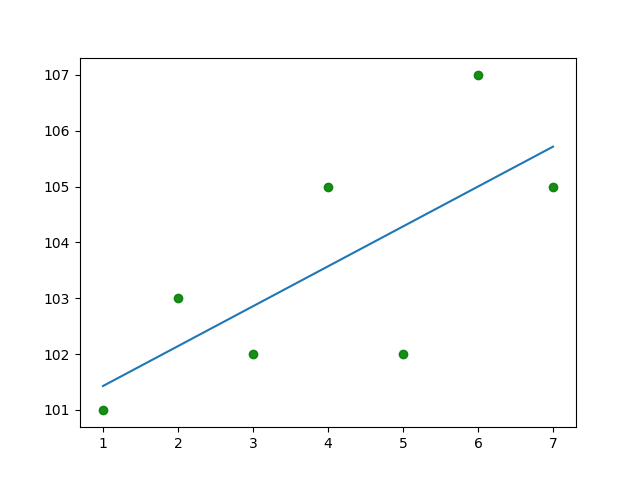# What is Linear Regression

Linear regression attempts to model the relationship between two variables by fitting a linear equation to observed data. One variable is considered to be an explanatory variable, and the other is considered to be a dependent variable. The case of one explanatory variable is called simple linear regression. For more than one explanatory variable, the process is called multiple linear regression.

Linear regression models are often fitted using the least squares approach.

If there appears to be no association between the proposed explanatory and dependent variables (i.e., the scatterplot does not indicate any increasing or decreasing trends), then fitting a linear regression model to the data probably will not provide a useful model. A valuable numerical measure of association between two variables is the correlation coefficient, which is a value between -1 and 1 indicating the strength of the association of the observed data for the two variables.

There are many names for a regression’s dependent variable. It may be called an outcome variable, criterion variable, endogenous variable, or regressand. The independent variables can be called exogenous variables, predictor variables, or regressors.

Linear regression using python

Following are the ways to do linear regression using python

1. statsmodels
2. scikit-learn
3. scipy

### Linear Regression using statsmodels

Here is sample code

and here is the output### Linear Regression using scikit-learn

Here is the code

and output of this code is as below### Linear Regression using scipy

Sample code

and outputIf you look at code, it seems finding linear regression using scipy is shortest and easiest to understand.

This site uses Akismet to reduce spam. Learn how your comment data is processed.